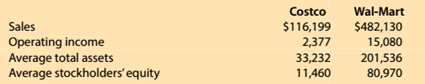Chapter 15, Problem 15.5.2MBA

Chapter
Section
Textbook Problem

Financial leverageCostco Wholesale Corporation (COST) and Wel-Mart Stroes Inc. (WMT)reported the following data (in milllions) for a recent year:Compute the return on stockholders equity. Round to one decimal place.

To determine

Concept Introduction:

Return on Equity:

Return on Equity is the rate of return earned by the Stockholders on their investment in the company. It is calculated with the help of following formula:

Return on Equity = Net IncomeAverage Stockholders Equity

The Average stock holder's equity calculated with the help of following formula:

Average stock holders equity=( Beginning stock holders equity + Ending stock holders equity)2

To Calculate:

The Return on Stockholder's equity

Explanation

The Return on Stockholder's equity is calculated as follows:

 $in Millions Costco Wal-Mart Operating Income (A)$ 2,377

Still sussing out bartleby?

Check out a sample textbook solution.

See a sample solution

The Solution to Your Study Problems

Bartleby provides explanations to thousands of textbook problems written by our experts, many with advanced degrees!

Get Started

Find more solutions based on key concepts